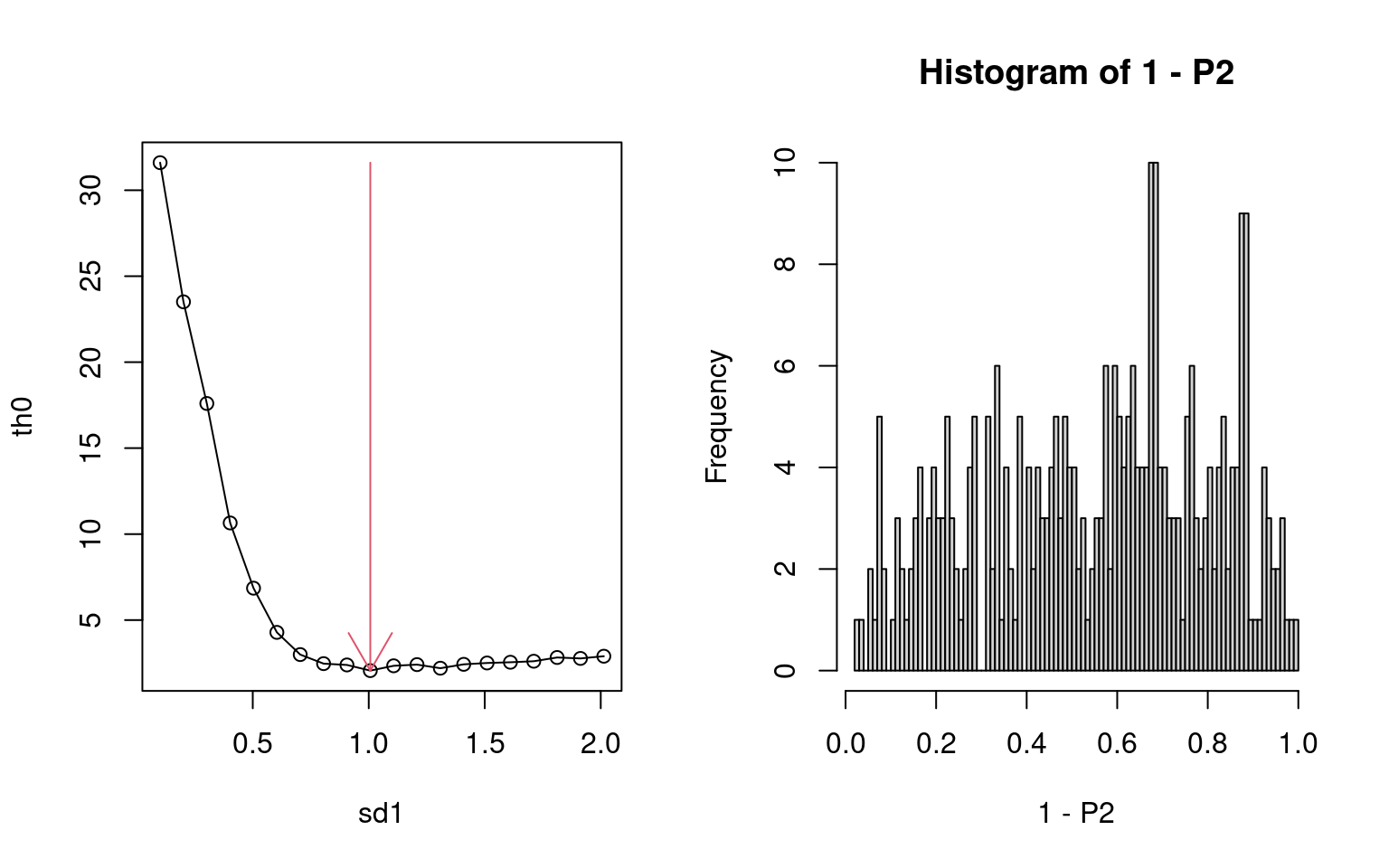# 1 Installation

if (!require("BiocManager", quietly = TRUE))
install.packages("BiocManager")
BiocManager::install("TDbasedUFE")

# 2 Introduction

library(TDbasedUFE)

Here I introduce how we can use TDbasedUFE to process real data.

# 3 Data Analyses

## 3.1 Gene expression.

Here is how we can process real data. First we prepare the data set from tximportdata package

require(GenomicRanges)
#>
#> Attaching package: 'BiocGenerics'
#> The following objects are masked from 'package:stats':
#>
#>     IQR, mad, sd, var, xtabs
#> The following objects are masked from 'package:base':
#>
#>     Filter, Find, Map, Position, Reduce, anyDuplicated, aperm, append,
#>     as.data.frame, basename, cbind, colnames, dirname, do.call,
#>     duplicated, eval, evalq, get, grep, grepl, intersect, is.unsorted,
#>     lapply, mapply, match, mget, order, paste, pmax, pmax.int, pmin,
#>     pmin.int, rank, rbind, rownames, sapply, setdiff, sort, table,
#>     tapply, union, unique, unsplit, which.max, which.min
#>
#> Attaching package: 'S4Vectors'
#> The following object is masked from 'package:utils':
#>
#>     findMatches
#> The following objects are masked from 'package:base':
#>
#>     I, expand.grid, unname
require(rTensor)
#>
#> Attaching package: 'rTensor'
#> The following object is masked from 'package:S4Vectors':
#>
#>     fold
library("tximport")
library("tximportData")
dir <- system.file("extdata", package="tximportData")
samples$condition <- factor(rep(c("A","B"),each=3)) rownames(samples) <- samples$run
samples[,c("pop","center","run","condition")]
#>           pop center       run condition
#> ERR188297 TSI  UNIGE ERR188297         A
#> ERR188088 TSI  UNIGE ERR188088         A
#> ERR188329 TSI  UNIGE ERR188329         A
#> ERR188288 TSI  UNIGE ERR188288         B
#> ERR188021 TSI  UNIGE ERR188021         B
#> ERR188356 TSI  UNIGE ERR188356         B
files <- file.path(dir,"salmon", samples$run, "quant.sf.gz") names(files) <- samples$run
#> Rows: 200401 Columns: 2
#> ── Column specification ────────────────────────────────────────────────────────
#> Delimiter: ","
#> chr (2): TXNAME, GENEID
#>
#> ℹ Use spec() to retrieve the full column specification for this data.
#> ℹ Specify the column types or set show_col_types = FALSE to quiet this message.
txi <- tximport(files, type="salmon", tx2gene=tx2gene)
#> 1 2 3 4 5 6
#> summarizing abundance
#> summarizing counts
#> summarizing length

As can be seen on the above, this data set is composed of six samples, which are divided into two classes, each of which includes three out of six samples. The number of features is 58288

dim(txi$counts) #>  58288 6 which is too many to execute small desktops, we reduce number of features to 10,000 txi[seq_len(3)] <- lapply(txi[seq_len(3)], function(x){dim(x);x[seq_len(10000),]}) Then we reformat count data, txi$counts, as a tensor, $$Z$$, whose dimension is $$10000 \times 3 \times 2$$ and HOSVD was applied to $$Z$$ to get tensor decomposition using function computeHosvd.

Z <- PrepareSummarizedExperimentTensor(matrix(samples$sample,c(3,2)), rownames(txi$abundance),array(txi$counts,c(dim(txi$counts),3,2)))
dim(attr(Z,"value"))
#>  10000     3     2
HOSVD <- computeHosvd(Z)
#>
|
|                                                                      |   0%
|
|=======================                                               |  33%
|
|===============================================                       |  67%
|
|======================================================================| 100%

Here 3 and 2 stand for the number of samples in each class and the number of classes, respectively. HOSVD includes output from HOSVD. Next, we need to decide which singular value vectors are used for the download analysis interactively. Since vignettes has no ability to store the output from interactive output from R, you have to input here as

input_all <- selectSingularValueVectorSmall(HOSVD,input_all= c(1,2)) #batch mode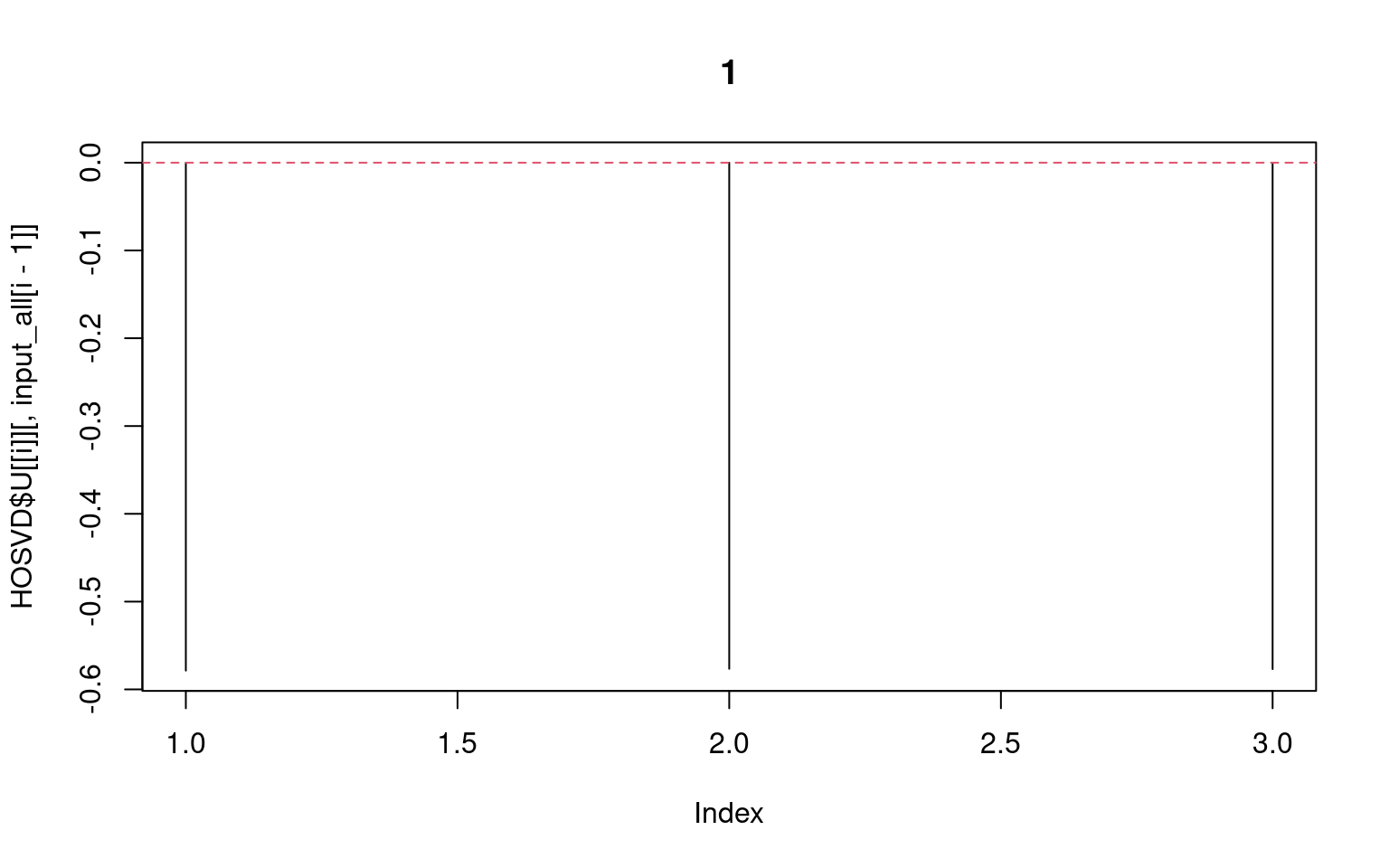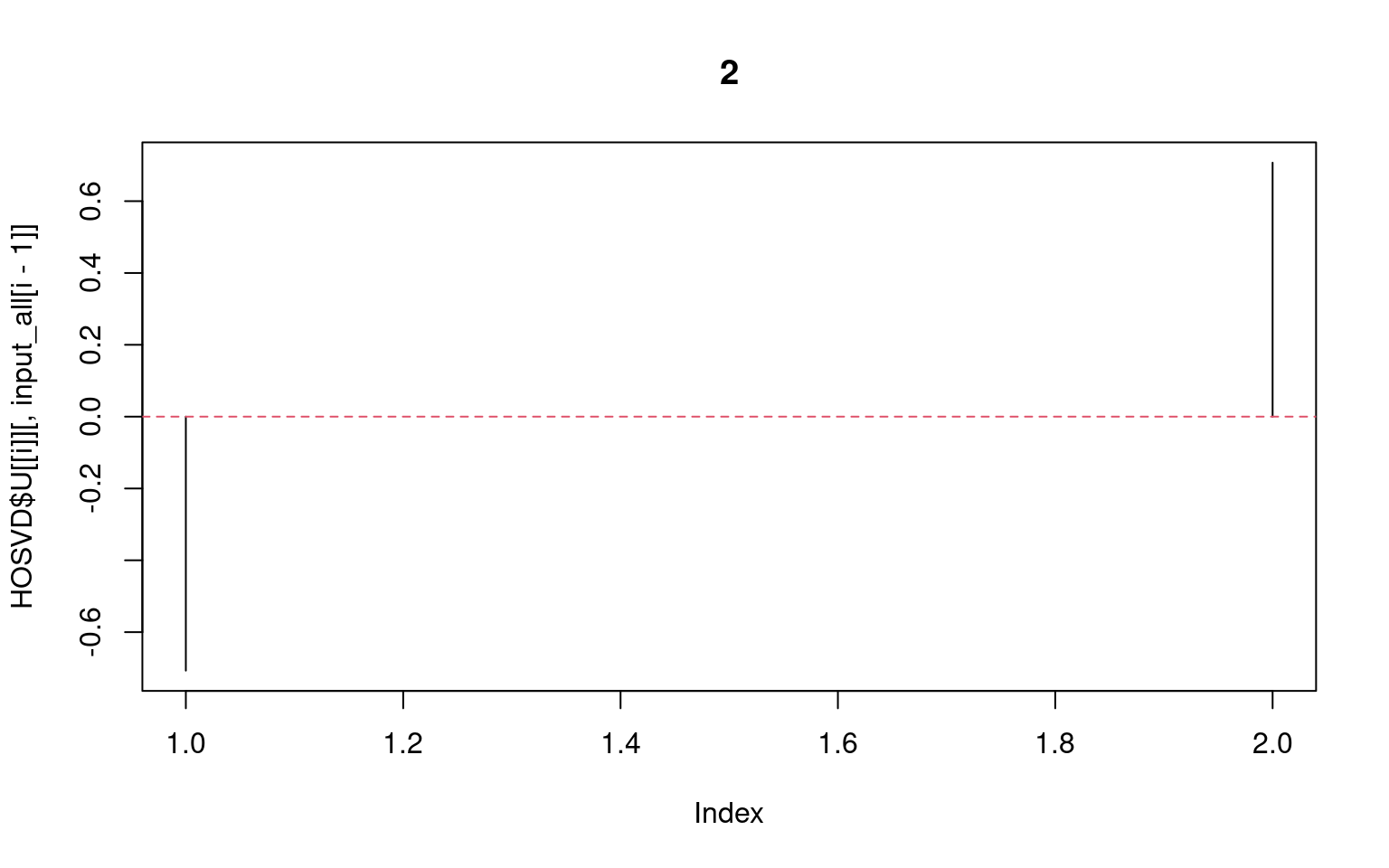in batch mode. Then you get the above graphic. In actual usage you can activate interactive mode as

input_all <- selectSingularValueVectorSmall(HOSVD)

In interactive mode, you can see “Next”, “Prev” and “Select” radio buttons by which you can select singular value vectors one by one interactively. Now 1 and 2 is entered in input_all, since these correspond to constant among three samples (AAA or BBB) and distinction between A and B, respectively.

Then we try to select features.

index <- selectFeature(HOSVD,input_all)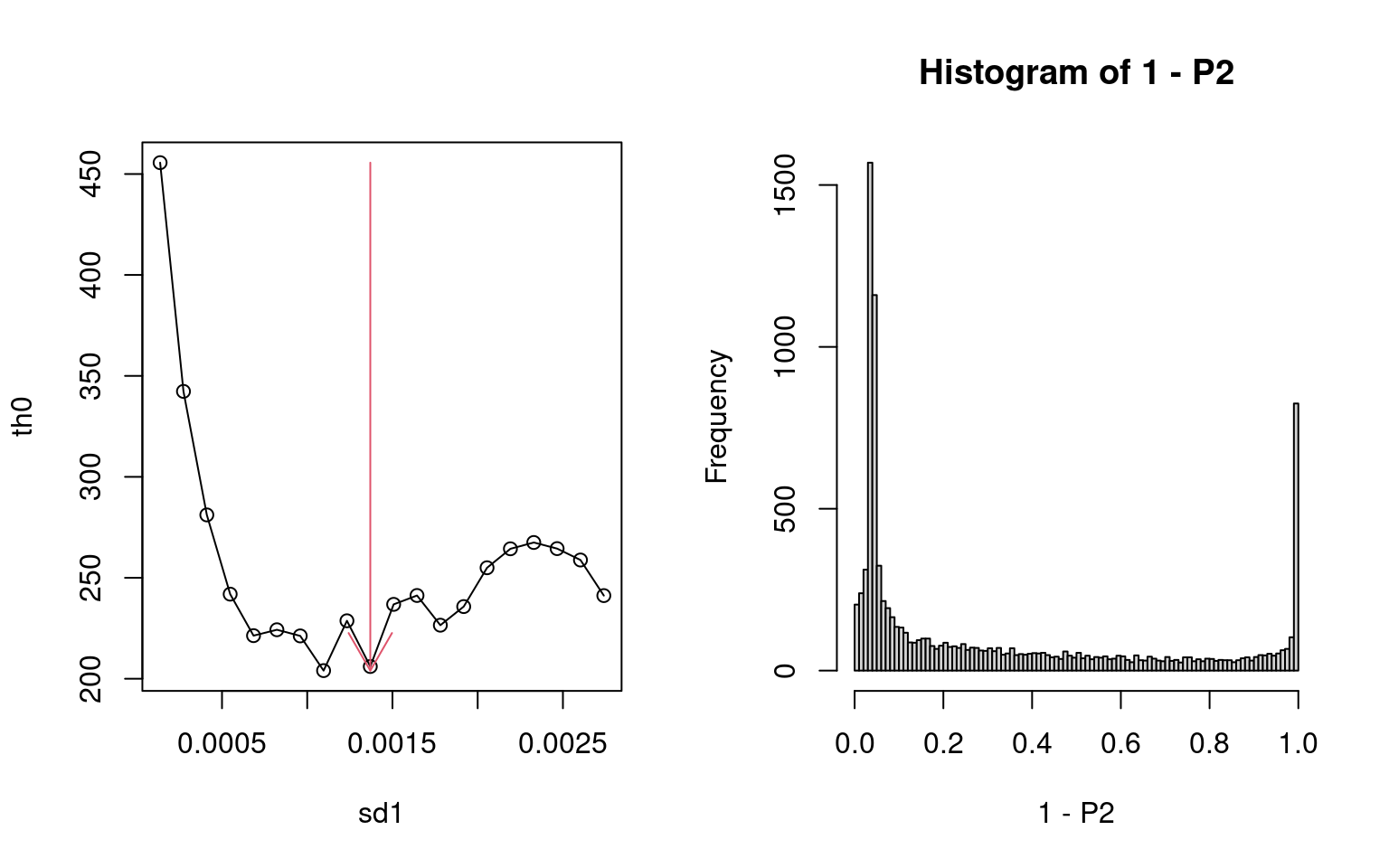We get the above graphic. The left one represents the dependence of “flatness” of histogram of $$P$$-values computed with assuming the null hypothesis. More or less it can select smallest value. Thus it is successful. The right one represents the histogram of 1-$$P$$-values. Peak at right end corresponds to genes selected (The peak at the left end does not have any meaning since they corresponds to $$P \simeq 1$$). Then we try to see top ranked features

head(tableFeatures(Z,index))
#>                Feature p value adjusted p value
#> 11   ENSG00000008988.9       0                0
#> 40   ENSG00000044574.7       0                0
#> 89  ENSG00000075618.17       0                0
#> 90  ENSG00000075624.13       0                0
#> 104 ENSG00000080824.18       0                0
#> 118 ENSG00000086758.15       0                0

These are associated with $$P=0$$. Thus, successful.

## 3.2 Multiomics data analysis

Next we try to see how we can make use of TDbasedUFE to perform multiomics analyses. In order that, we prepare data set using MOFAdata package as follows.

require(MOFAdata)
data("CLL_data")
data("CLL_covariates")
help(CLL_data)

CLL_data is composed of four omics data,

• Drugs: viability values in response to 310 different drugs and concentrations
• Methylation: methylation M-values for the 4248 most variable CpG sites
• mRNA: normalized expression values for the 5000 most variable genes
• Mutations: Mutation status for 69 selected genes

each of which was converted to squared matrix (i. e., liner kernel(Taguchi and Turki 2022))and they are bundle as one tensor using PrepareSummarizedExperimentTensorSquare function, to which HOSVD is applied as follows.

Z <- PrepareSummarizedExperimentTensorSquare(
sample=matrix(colnames(CLL_data$Drugs),1), feature=list(Drugs=rownames(CLL_data$Drugs),
Methylation=rownames(CLL_data$Methylation), mRNA=rownames(CLL_data$mRNA),Mutations=rownames(CLL_data\$Mutations)),
value=convertSquare(CLL_data),sampleData=list(CLL_covariates[,1]))
HOSVD <- computeHosvdSqure(Z)
#>
|
|                                                                      |   0%
|
|=======================                                               |  33%
|
|===============================================                       |  67%
|
|======================================================================| 100%

CLL_covariate is labeling information, among which we employed the distinction between male (m) and female (f).

table(CLL_covariates[,1])
#>
#>   f   m
#>  79 121
cond <- list(attr(Z,"sampleData")[],attr(Z,"sampleData")[],seq_len(4))

Since a tensor is a bundle of liner kernel, the first two singular value vectors are dedicated to samples and the third (last) one is dedicated to four omics classes (Drugs, Methylation, mRNA and mutations). In order to select which singular value vectors are employed, we execute selectFeatureSquare function in batch mode as follows

input_all <- selectSingularValueVectorLarge(HOSVD,cond,input_all=c(8,1))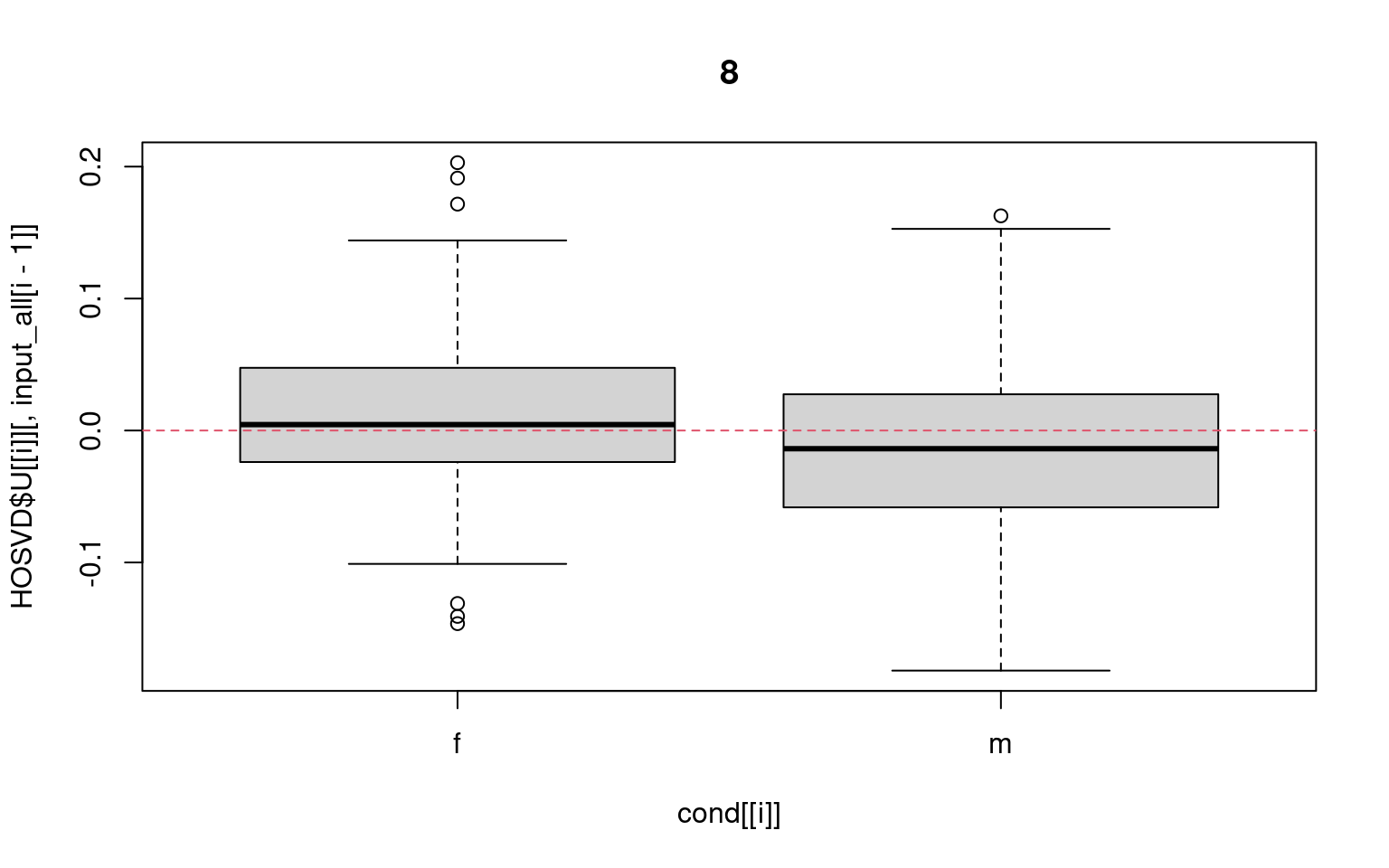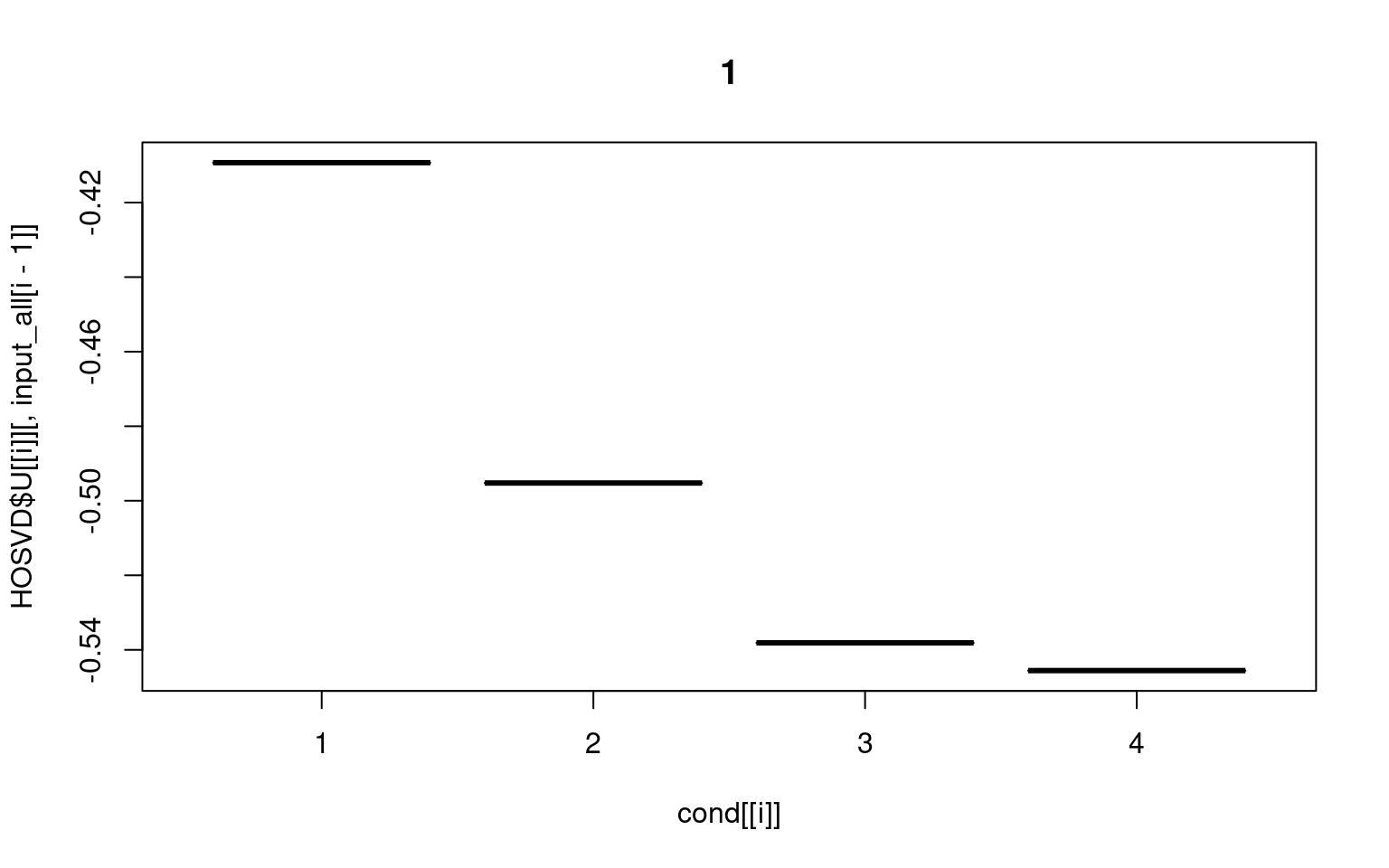In actual usage you can activate interactive mode as

input_all <- selectSingularValueVectorLarge(HOSVD,cond)

and can select the eight singular value vetor for samples that represents distinction between male and female and the first singular value vectors to four omics categories that represents the commoness between four omics data (i.e., constant values for four omics data).

Now we come to the stage to select features. Since there are four features, we need to optimize SD for each of them. Try to execute selectFeatureSquare in a batch mode as follows.

index <- selectFeatureSquare(HOSVD,input_all,CLL_data,
de=c(0.3,0.03,0.1,0.1),interact=FALSE) #for batch mode